--> --> Understanding the Seismic Wavelet; Steven G. Henry; Search and Discovery Article #40028 (2001)

Understanding the Seismic Wavelet*

By

Steven G. Henry1

Search and Discovery Article #40028 (2001)

1Geolearn, Houston, TX ([email protected])

*Adapted for online presentation from two articles by same author, entitled “Catch the (Seismic) Wavelet” in Geophysical Corner, AAPG Explorer, March, 1997, and “Zero Phase Can Aid Interpretation” in Geophysical Corner, AAPG Explorer, April, 1997. Appreciation is expressed to the author and to M. Ray Thomasson, former Chairman of the AAPG Geophysical Integration Committee, and Larry Nation, AAPG Communications Director, for their support of this online version.

 uComparison of different methods of deconvolution                                                           uComparison of different methods of deconvolution                                                       uComparison of different methods of deconvolution                                                           uComparison of different methods of deconvolution                                                           uComparison of different methods of deconvolution                                                           uComparison of different methods of deconvolution                                                           uComparison of different methods of deconvolution Summary Statement The seismic wavelet is the link between seismic data (traces) on which interpretations are based and the geology (reflection coefficients) that is being interpreted, and it must be known to interpret the geology correctly. However, it is typically unknown, and assumed to be both broad band and zero phase. Providing this broad band, zero phase wavelet is the processing goal of deconvolution. Unfortunately, this goal is rarely met and the typical wavelet that remains in fully processed seismic data is mixed-phase. Differences in mixed-phase wavelets result in mis-ties and often incorrect interpretations. The purpose of this article is to show interpreters that significant improvements in seismic data quality and, correspondingly, their interpretations of those data are easily obtainable by converting from mixed-phase to zero phase wavelets. Figure Captions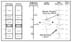Figure 1: Lithologic boundaries define a Reflection Coefficient series, which when convolved (*) with the field wavelet results in a simulated raw field trace. Interpreting the highest amplitude event (2.5 seconds) as the reservoir sand would be wrong. This mixed-phase wavelet provides a distorted image of the actual geology.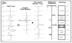Figure 2: When the field wavelet is known, deterministic deconvolution is able to produce a processed trace that contains the desired broad band zero phase wavelet. Note, the highest amplitude in the processed trace is now associated with the largest Reflection Coefficient at the top sand.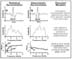Figure 5. These wavelets have been extracted from the seismic in Figures 7 and 8 using the well reflection Coefficients Statistical Deconvolution (a) commonly produces mixed-phase wavelets. The broad band - zero phase processing goal of deconvolution has been met using Deterministic Deconvolution (b).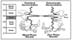Figure 6. The mixed-phase wavelet (Figure 5a) has the character of “Pinocchio with a beer-gut.”  Each reflector has a sharp nose followed by a low-frequency “beer-gut.”  The zero phase wavelet (Figure 5b) has all the energy moved up to Pinocchio’s nose. Reflectors lacking the wavelet’s beer-gut more clearly image the subsurface geology.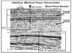Figure 7. Seismic data identical to Figure 8 other than the method of deconvolution, Statistical. This seismic data contains the mixed-phase wavelet of Figure 5a. The “Pinocchio with a “beer-gut” character can be seen as the trailing low frequencies beneath the high amplitude reflectors. Mixed-phase wavelets reduces the ability to accurately resolve the subsurface geology.  Click here for sequence of Figures 7  and 8.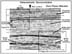Figure 8. Seismic data identical to Figure 7 other than method of deconvolution, Deterministic. This seismic data contains the desired zero phase wavelet (Figure 5b). Note that reflectors are sharper and lack the low-frequency “beer-gut” of the mixed- phase wavelet. In general reflector continuity, fault breaks, and stratigraphic relationships are significantly improved. Click here for sequence of Figures 7  and 8.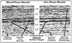Figure 9. Enlargement of corresponding parts in upper left of Figures 7 and 8, showing significantly different seismic images from the same subsurface geology. The zero phase (b) seismic data not only “looks” better, but also provides a more accurate image. Mixed-phase wavelets distort the seismic image and can lead to incorrect interpretations.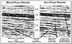Figure 10. Enlargement from corresponding parts in lower right of Figures 7 and 8 showing significant improvements in the definition of faults are seen on the zero phase seismic data (b). “Pinocchio’s beer-gut” in the mixed-phase seismic data (a) hangs over the faults, distorting the image. Reflector continuity and stratigraphic relationships are also improved in the seismic data containing the zero phase wavelet. Return to top. Goals of Deconvolution Seismic data can provide a remarkably good image of the subsurface. However, without knowing the seismic wavelet, there are many equally valid surface geologic interpretations of the actual subsurface geology. The seismic wavelet is the filter through which geology is viewed when interpreting the image provided by seismic data. The common assumption that seismic data contain a broad band - zero phase wavelet is nearly always wrong. The majority of mis-tie problems between seismic and synthetics, seismic to seismic of different vintages, and many of the misinterpretations based on modeling (lithology prediction, trace attributes, AVO, etc.) are the result of mixed-phase wavelets remaining in fully processed seismic data. The convolutional model is useful for understanding how changes in rock properties (velocity and density) result in the waveform changes observed in seismic data. At lithologic boundaries the magnitude of change (reflection coefficient) in these rock properties determines how much of the wavelet’s energy is reflected to the surface. In acquiring seismic data (Figure 1), the subsurface is illuminated with sonic energy (field wavelet), which is reflected from these acoustic boundaries and recorded at the surface as a raw field trace. Where lithologic boundaries are widely separated, the field wavelet can be seen “hanging” below the reflector at 2.2 seconds (Figure 1). When boundaries are more closely spaced (2.3-2.5 seconds), the wavelet is not as easily seen due to the wavelets being summed together. This summing is also known as convolution. The convolutional model states that all seismic traces are the result of convolving (summing) the wavelet with the reflection coefficient series. In Figure 1, the raw field trace images the desired geology (lithologic boundaries = reflection coefficients), but it is through the complex filter (convolution) of the field wavelet. Exploring for the sand in Figure 1 and assuming the wavelet is broad band - zero phase, the sand should be the largest peak. The largest peak, however, is at 2.5 seconds, due to the field wavelet not being zero phase. When the wavelet in the seismic trace is unknown, the geology is unknown. Interpretations are not made on raw field traces, but even on processed seismic traces, the wavelet must be known to more correctly interpret the geology. The seismic processing procedure designed to convert the field wavelet to the desired broad band - zero phase wavelet is deconvolution. The two common methods of deconvolution are deterministic deconvolution and statistical deconvolution. Deterministic deconvolution can be applied when the field wavelet is known (measured and/or modeled). As shown in Figure 2, when the wavelet is known, an inverse can be determined and the field trace deconvolved to contain the desired zero phase wavelet. When processed traces contain a zero phase wavelet, increases in rock velocity (shale to sand) will result in peaks (positive reflection coefficients).  More typically, the field wavelet is unknown and statistical deconvolutions must be used. Statistical deconvolutions must make assumptions about both the wavelet and the reflection coefficient series. The most common assumption is that the wavelet is minimum phase and that the reflection coefficient series is random. Return to top. Over 90 percent of all seismic data are processed assuming minimum phase. Examples of a few of the more popular minimum phase deconvolutions include Spiking, Gapped, Predictive, and Adaptive. Unfortunately, most field wavelets are not minimum phase, and that basic assumption is not met. Using minimum phase deconvolutions typically results in processed traces that contain mixed-phase rather than the desired zero phase wavelets. A wavelet extracted deterministically from seismic data (using the known reflection coefficient series from the well) that had been deconvolved assuming minimum phase is shown in Figure 3. Note that this wavelet is not zero phase (phase spectrum: constant zero value for all frequencies) but is mixed phase (non-linear, variable for all frequencies). In describing mixed-phase wavelets, it is useful to group frequency into bands in which a linear fit can be extrapolated to the phase axis (phase spectrum). For the wavelet in Figure 3, the higher frequencies (20-65 Hertz) have a phase of -90 degrees, while the lower frequencies (5-20 Hertz) have a phase of +60 degrees. The description of this mixed-phase wavelet is interpretive, and could be described differently by using other frequency bands. Using the bands shown in Figure 3, with most of the power (amplitude spectrum squared) in the 20-65 Hertz band, this wavelet has the phase characteristic of -90 degrees (trough-peak). An important ramification of mixed-phase wavelets is that their peak-trough relationships will change with depth due to the effects of earth filtering. In the case of the wavelet shown in Figure 3, shallow in the section where the earth has not filtered the higher frequences (maximum power 20-60 Hertz), positive reflectors (low to high velocity) will display -90 degrees (trough-peak). Deep in the section where the high frequencies have been attenuated (dashed line in the amplitude spectra, Figure 3), the wavelet will appear with the phase characteristics of the lower frequencies and will have a phase of +60 degrees (peak-trough). Mixed-phase wavelets are the most common wavelets found in seismic data and can have a strong affect on interpretations. This is shown in Figure 4 by filtering back (5-20 Hertz) the processed wavelet to illustrate strong earth filtering. The identical reservoir sandstone would appear as a trough-peak (-90 degrees) shallow (1.0 seconds) in the section and as a peak-trough (+60 degrees) deeper (3.0 seconds) in the section. This change in the peak-trough relationship due to earth filtering is commonly observed when comparing constant phase synthetics (from well logs) to seismic data. Typically this problem is compensated by applying a bulk time shift (linear phase shift) and changing the constant phase wavelet used in making the synthetic. Combining these corrections will approximate the curved shape of the mixed-phase (Figure 3) with a single sloping line (time shift) that intersects the phase axis at the desired constant phase. A different time shift and constant phase is required to match the curve deeper in the section due to the earth’s absorption of higher frequencies. Due to the mixed-phase wavelet, peak-trough relationship change as a function of earth absorption (Figure 4), and interpretations based on amplitudes, AVO, attributes, etc., are likely to be incorrect. The solutions to these problems are to convert the mixed-phase wavelet to zero phase in seismic processing or to extract the mixed-phase wavelet, know its effects, and use it when modeling. When interpreting seismic data, it is important to realize that the actual subsurface geology is always being viewed through the filter of the seismic wavelet. Although deconvolution is designed to provide a broad band - zero phase wavelet, this goal is typically not met, and most seismic data contain mixed-phase wavelets. Mixed-phase wavelets affect interpretations and, as noted below, degrade the quality of seismic data. Return to top. Comparison of Seismic Data After Different Methods of Deconvolution The wavelets shown in Figure 5 were extracted from the seismic data in Figures 7 and 8. Both sets of seismic data were processed identically, other than the method of deconvolution (statistical-minimum phase vs. deterministic). The wavelets were extracted deterministically, by cross-correlating (Wiener-Levinson filter) seismic traces with the known geology derived from the subsurface well reflection coefficient series. At first glance, most interpreters would be content having either of these “spike-like” wavelets convolved on the subsurface reflectors. The primary difference between these wavelets is that statistical deconvolution has resulted in a mixed-phase wavelet (Figure 5 – phase spectrum), whereas the deterministic deconvolution provided the desired broad band, zero phase wavelet. These seemingly minor differences in the phase spectra (Figure 5) have a significant effect on the seismic data’s overall quality. Although the “quality” of seismic data has always been in the “eye of the beholder,” the conversion from mixed-phase to zero phase provides a more accurate image of the subsurface geology. The reason for the improved accuracy is illustrated in Figure 6. The extracted wavelets (Figure 5) have been convolved on a single reflector (Top Sand). Note that the mixed-phase wavelet has sharp “Pinocchio-like” nose at the top of the sand, but is followed by a low-frequency “beer-gut.” When seismic data contains a mixed-phase wavelet, each reflector has this “Pinocchio with a beer-gut” character. Neighboring reflectors deeper in the section (base sand) are phase rotated and lose amplitude as their “noses” are summed with the “beer-guts” from above. The zero phase wavelet’s sharp “nose” provides a clear image of the top sand, and its “flat belly” does not interfere with neighboring reflectors. The comparison of the seismic data in Figures 7 and 8 illustrate typical improvements that are easily obtainable by converting from mixed-phase to zero phase. Both seismic sections “look” good, and visual advantages can be found in each. In general, however, the seismic data containing the zero phase wavelet (Figure 8) have a better overall reflector continuity, better fault definition and more easily identified stratigraphic relationships. Mixed-phase wavelets may occasionally tune to enhance a particular reflector (Figure 7 – increased continuity), but the overall quality of mixed-phase data is lower. The seismic data comparison for the shallower section is shown in Figure 9. Return to top. Due to minor earth absorption, the majority of the wavelet’s phase characteristics, as noted above, are derived from the higher frequencies (20-65 Hertz). The lower frequencies, although contributing less, still influence the character of the reflectors. The non-zero phase components (5-20 Hertz) of the mixed-phase wavelet (Figure 9a) can be seen distorting the image of the geology. These two images (Figure 9a and b) of the same geology would likely result in different interpretations. For example, laterally discontinuous reflectors within the high amplitude package (2.2-2.3 seconds) appear with different seismic character and even in different locations. Interpretation of them (channel sands? carbonate mounds?) and their position relative to the deeper high amplitude reflector (within/below--Figure 9a, or above--Figure 9b) are in question. Knowing that mixed-phase wavelets distort the seismic image, the interpretation should be made from the zero phase seismic data. In general, the zero phase wavelet provides a much sharper (broad band - zero phase) image of the subsurface geology. Stratigraphic relationships above 2.0 seconds (Figure 9) are more clearly defined, and reflector continuity in general (especially 2.6 seconds) is improved. Deeper in the section (Figure 10), as the earth’s filtering of the higher frequencies increases, the lower frequencies contribute more strongly to the wavelet’s phase characteristic (as described above). In the mixed-phase seismic data, the non-zero (135 degrees) low-frequency (5-20 Hertz) components begin contributing more. This adds to the distortion seen shallow in the section (Figure 9), further reducing the ability to image the geology accurately. Absorption also affects the zero phase wavelet, reducing high frequencies (narrowing the bandwidth) and thereby stretching out the wavelet. However, since all frequencies (5-65 Hertz) are zero phase, the seismic image provides an accurate representation of the geology.  The most striking improvement seen in the zero phase seismic data (Figure 10b) is the ability to define faults more accurately. The mixed-phase wavelet’s “beer-gut,” which has grown with depth due to absorption (loss of high frequencies), is hanging in the fault zones. “Noses” on the other side of the faults are smeared out by the “beer-guts” from above. Other significant improvements seen in the zero phase seismic data are the improved continuity of reflectors and the imaging of geologic details needed for stratigraphic interpretations. The zero phase wavelet, as shown in these examples, provides a more accurate image of the subsurface geology than the mixed-phase wavelet. Conclusion Significant improvements in the quality of seismic data are shown here to be easily obtainable when wavelets are converted from mixed-phase to zero phase by extracting the wavelet and filtering the data. This added effort to provide seismic data that meets the zero phase assumption will improve the accuracy of the interpreted subsurface geology and provide the correct input to many software packages (Attributes, Amplitudes, AVO) used to reduce exploration risks. Return to top.